International
Tables for
Crystallography
Volume A1
Symmetry relations between space groups
Edited by Hans Wondratschek and Ulrich Müller

International Tables for Crystallography (2006). Vol. A1, ch. 1.3, pp. 24-25   | 1 | 2 |

## Section 1.3.2. Crystallographic orbits and Wyckoff positions

Ulrich Müllera*

aFachbereich Chemie, Philipps-Universität, D-35032 Marburg, Germany
Correspondence e-mail: mueller@chemie.uni-marburg.de

### 1.3.2. Crystallographic orbits and Wyckoff positions

| top | pdf |

The set of symmetry-equivalent sites in a space group is referred to as a (crystallographic point) orbit (Koch & Fischer, 1985; Wondratschek, 1976, 1980, 2005; also called point configuration). If the coordinates of a site are completely fixed by symmetry (e.g.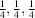), then the orbit is identical with the corresponding Wyckoff position of that space group (in German Punktlage). However, if there are one or more freely variable coordinates (e.g. z in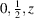), the Wyckoff position comprises an infinity of possible orbits; they differ in the values of the variable coordinate(s). The set of sites that are symmetry equivalent to, say,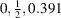make up one orbit. The set corresponding to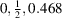belongs to the same Wyckoff position, but to another orbit (its variable coordinate z is different).

The Wyckoff positions of the space groups are listed in Volume A of International Tables for Crystallography (2005). They are labelled with letters a, b,, beginning from the position having the highest site symmetry. A Wyckoff position is usually given together with the number of points belonging to one of its orbits within a unit cell. This number is the multiplicity listed in Volume A, and commonly is set in front of the Wyckoff letter. For example, the denomination 4c designates the four symmetry-equivalent points belonging to an orbit c within the unit cell.

In many space groups, for some Wyckoff positions there exist several Wyckoff positions of the same kind that can be combined to form a Wyckoff set [called a Konfigurationslage by Koch & Fischer (1975)]. They have the same site symmetries and they are mapped onto one another by the affine normalizer of the space group (Koch & Fischer, 1975; Wondratschek, 2005).

#### Example 1.3.2.1.

In space group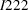, No. 23, there are six Wyckoff positions with the site symmetry 2: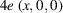,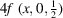on twofold rotation axes parallel to a,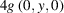,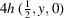on twofold rotation axes parallel to b,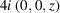,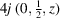on twofold rotation axes parallel to c.

They are mapped onto one another by the affine normalizer of, which is isomorphic to, No. 221. These six Wyckoff positions make up one Wyckoff set.

However, in this example the positions 4e, 4f vs. 4g, 4h vs. 4i, 4j, being on differently oriented axes, cannot be considered to be equivalent if the lattice parameters are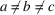. The subdivision of the positions of the Wyckoff set into these three sets is accomplished with the aid of the Euclidean normalizer of the space group.

The Euclidean normalizer is that supergroup of a space group that maps all equivalent symmetry elements onto one another without distortions of the lattice. It is a subgroup of the affine normalizer (Fischer & Koch, 1983; Koch et al., 2005). In Example 1.3.2.1.(space group), the positions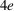and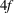are equivalent under the Euclidean normalizer (and so are 4g, 4h and also 4i, 4j). The Euclidean normalizer of the space groupis, No. 47, with the lattice parameters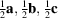(if). If the origin of a space group is shifted, Wyckoff positions that are equivalent under the Euclidean normalizer may have to be interchanged. The permutations they undergo when the origin is shifted have been listed by Boyle & Lawrenson (1973). An origin shift of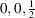will interchange the Wyckoff positionsandas well as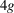and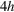of.

#### Example 1.3.2.2.

In the space group, No. 225, the orbits of the Wyckoff positions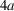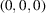and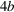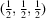are equivalent under the Euclidean normalizer. The copper structure can be described equivalently either by having the Cu atoms occupy the positionor the position. If we take Cu atoms in the positionand shift the origin fromto, then they result in the position.

Unique relations exist between the Wyckoff positions of a space group and the Wyckoff positions of any of its subgroups (Billiet et al., 1978; Wondratschek, 1993; Wondratschek et al., 1995). Given the relative positions of their unit cells (axes transformations and relative origin positions), the labels of these Wyckoff positions are unique.

#### Example 1.3.2.3.

In diamond, the carbon atoms occupy the orbit belonging to the Wyckoff position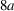of the space group, No. 227. Sphalerite (zinc blende) crystallizes in the maximal subgroup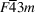, No. 216, of. With the transition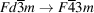the Wyckoff positionsplits into the positionsand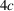of. These are two symmetry-independent positions that allow an occupation by atoms of two different elements (zinc and sulfur). In this example, all of the positions retain the site symmetryand each Wyckoff position comprises only one orbit.

### References

Billiet, Y., Sayari, A. & Zarrouk, H. (1978). L'asso­ciation des familles de Wyckoff dans les changements de repère conventionnel des groupes spatiaux et les passages aux sous-groupes spatiaux. Acta Cryst. A34, 811–819.Google Scholar
Boyle, L. L. & Lawrenson, J. E. (1973). The origin dependence of the Wyckoff site description of a crystal structure. Acta Cryst. A29, 353–357.Google Scholar
Fischer, W. & Koch, E. (1983). On the equivalence of point configurations due to Euclidean normalizers (Cheshire groups) of space groups. Acta Cryst. A39, 907–915.Google Scholar
International Tables for Crystallography (2005). Vol. A, Space-group symmetry, edited by Th. Hahn, 5th ed. Heidelberg: Springer. (Abbreviated IT A.)Google Scholar
Koch, E. & Fischer, W. (1975). Automorphismen­grup­pen von Raum­grup­pen und die Zuordnung von Punktlagen zu Kon­fi­gu­ra­tions­lagen. Acta Cryst. A31, 88–95.Google Scholar
Koch, E. & Fischer, W. (1985). Lattice complexes and limiting complexes versus orbit types and non-characteristic orbits: a comparative discussion. Acta Cryst. A41, 421–426.Google Scholar
Koch, E., Fischer, W. & Müller, U. (2005). Normalizers of space groups and their use in crystallography. International tables for crystallography, Vol. A, Space-group symmetry, edited by Th. Hahn, Part 15. Heidelberg: Springer.Google Scholar
Wondratschek, H. (1976). Extraordinary orbits of space groups. Theoretical considerations. Z. Kristallogr. 143, 460– 470.Google Scholar
Wondratschek, H. (1980). Crystallographic orbits, lattice complexes, and orbit types. MATCH Comm. Math. Chem. 9, 121–125.Google Scholar
Wondratschek, H. (1993). Splitting of Wyckoff positions (orbits). Mineral. Petrol. 48, 87–96.Google Scholar
Wondratschek, H. (2005). Special topics on space groups. International tables for crystallography, Vol. A, Space-group symmetry, edited by Th. Hahn, Part 8. Heidelberg: Springer.Google Scholar
Wondratschek, H., Müller, U., Aroyo, M. I. & Sens, I. (1995). Splitting of Wyckoff positions (orbits). II. Group–subgroup chains of index 6. Z. Kristallogr. 210, 567–573.Google Scholar